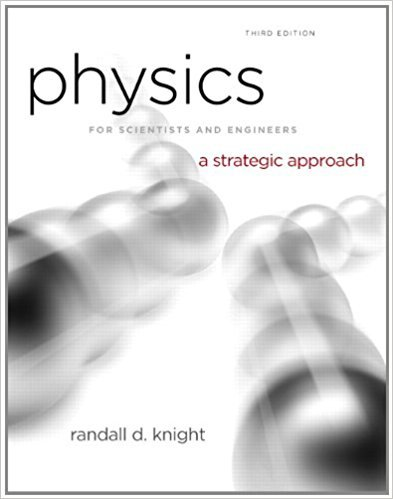×
Log in to StudySoup
Get Full Access to Physics For Scientists And Engineers: A Strategic Approach With Modern Physics - 3 Edition - Chapter 14 - Problem 79cp
Join StudySoup for FREE
Get Full Access to Physics For Scientists And Engineers: A Strategic Approach With Modern Physics - 3 Edition - Chapter 14 - Problem 79cp

Already have an account? Login here
×
Reset your password

# a. A mass m oscillating on a spring has period T. SupposeISBN: 9780321740908 69

## Solution for problem 79CP Chapter 14

Physics for Scientists and Engineers: A Strategic Approach with Modern Physics | 3rd Edition

• Textbook Solutions
• 2901 Step-by-step solutions solved by professors and subject experts
• Get 24/7 help from StudySoup virtual teaching assistantsPhysics for Scientists and Engineers: A Strategic Approach with Modern Physics | 3rd Edition

4 5 1 244 Reviews
19
1
Problem 79CP

Problem 79CP

a. A mass m oscillating on a spring has period T. Suppose the mass changes very slightly from m to m + ∆m, where ∆m ≪ m. Find an expression for ∆T, the small change in the period. Your expression should involve T, m, and ∆m but not the spring constant.

b. Suppose the period is 2.000 s and the mass increases by 0.1 %. What is the new period?

Step-by-Step Solution:

Solution 79 CP

Step 1 of 4

We need to derive the expression forWe are required to calculate the time period

Step 2 of 4

Step 3 of 4

##### ISBN: 9780321740908

The full step-by-step solution to problem: 79CP from chapter: 14 was answered by , our top Physics solution expert on 08/30/17, 04:34AM. The answer to “a. A mass m oscillating on a spring has period T. Suppose the mass changes very slightly from m to m + ?m, where ?m ? m. Find an expression for ?T, the small change in the period. Your expression should involve T, m, and ?m but not the spring constant.________________b. Suppose the period is 2.000 s and the mass increases by 0.1 %. What is the new period?” is broken down into a number of easy to follow steps, and 69 words. Since the solution to 79CP from 14 chapter was answered, more than 256 students have viewed the full step-by-step answer. Physics for Scientists and Engineers: A Strategic Approach with Modern Physics was written by and is associated to the ISBN: 9780321740908. This full solution covers the following key subjects: period, mass, spring, suppose, Expression. This expansive textbook survival guide covers 17 chapters, and 1439 solutions. This textbook survival guide was created for the textbook: Physics for Scientists and Engineers: A Strategic Approach with Modern Physics, edition: 3.

Unlock Textbook Solution

Enter your email below to unlock your verified solution to:

a. A mass m oscillating on a spring has period T. Suppose